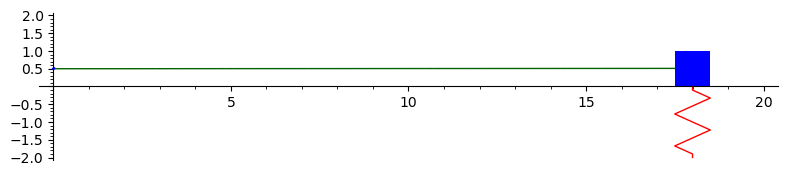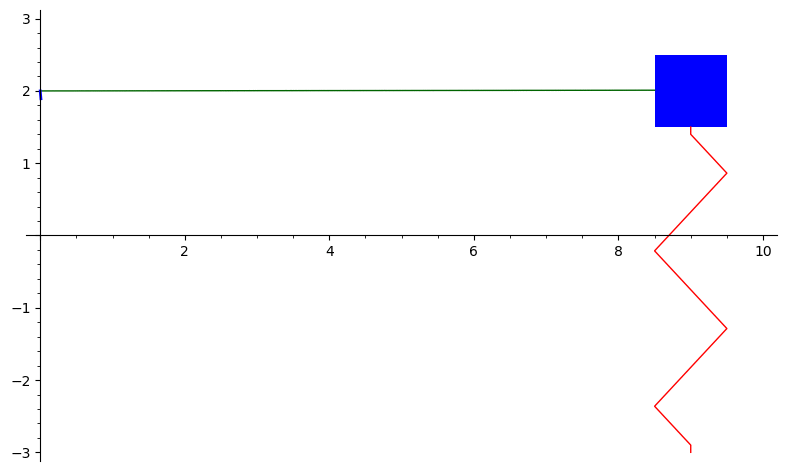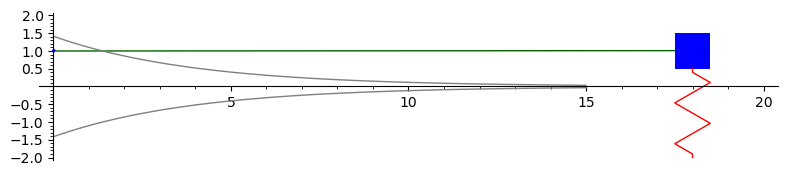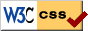# Notes on Diffy Qs - SAGE demonstrations for section 2.4

On this page the animations are prerendered. There is no reason to run the SAGE code unless you changed it. These animations take SAGE long time to draw. If you wish to experiment look for the 'srange' in the code to make it into fewer frames, the syntax is srange(start,end,step)'. In the code given, the steps are on purpose bigger than what was used to produce the animations, if you want to replicate the animations exactly you have to reduce the step size.

## Undamped motion

The graph below is the solution to $x'' + 4x = 0$ with initial conditions $x(0) = 0.5$ and $x'(0) = 1$. This represents the undamped motion of a mass on a spring. An animation is drawn of the mass on the spring. The solution is $x(t) = 0.5 \cos(2t) + 0.5 \sin(2t)$. To plot something different modify the solution below.Here is the SAGE code to produce this animation:

## Overdamped motion

The graph below is the solution to $2x'' + 7x' + 3x = 0$ with initial conditions $x(0) = 2$ and $x'(0) = -11$. This represents overdamped motion of a mass on a spring. The solution is $x(t) = 4e^{-3t} - 2e^{-0.5t}$.Here is the SAGE code to produce this animation:

## Underdamped motion

The graph below is the solution to $16x'' + 8x' + 63x = 0$ with initial conditions $x(0) = 1$ and $x'(0) = 1.75$. This represents underdamped motion of a mass on a spring. Here we also plot the envelop curves.Here is the SAGE code to produce this animation:

The code is mainly due to Ryan Burkhart, it was then slightly modified by Jiří Lebl.